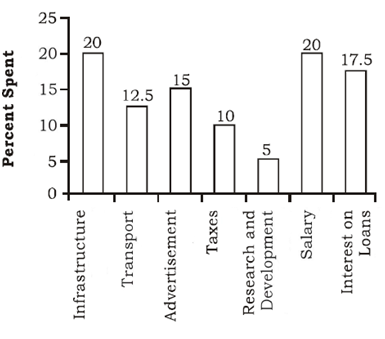# SSC CGL 2018 Practice Test Papers | Quantitative Aptitude (Day-37)

Dear Aspirants, Here we have given the Important SSC CGL Exam 2018 Practice Test Papers. Candidates those who are preparing for SSC CGL 2018 can practice these questions to get more confidence to Crack SSC CGL 2018 Examination.

[WpProQuiz 3286]

Click “Start Quiz” to attend these Questions and view Explanation

1) A student wrote 18 distinct numbers on a blackboard. The average of these numbers is 15. If the least of them was erased then the average of the remaining numbers would be 0.5 more. Find the least of them.

a) 5

b) 7

c) 5.5

d) 6.5

2) Three vessels contain milk solutions with concentrations of milk as 0.2, 0.4 and 0.5 respectively. Solution of three liters from the first, five liters from the second and two liters from the third are mixed. What is the resultant ratio of milk and water in the resultant mixture?

a) 9: 16

b) 8: 17

c) 2:3

d) 3:1

3) Simplify: 25 – 12 + 155 + 9 =? + 160

a) 13

b) 14

c) 17

d) 16

4) The sum of three consecutive integers is 1593. What is the value of 1/8 times of the largest number of three consecutive numbers?

a) 66.5

b) 61.5

c) 51.75

d) 52

5) The LCM and HCF of and two numbers is 180 and 15 respectively. If one number is 45 then what is 200% of the other number?

a) 100

b) 120

c) 300

d) 90

6) If 22x+8 = 86x then what is the value of x?

a) 3/2

b) 5

c) 1/2

d) 2

7) The difference between areas of a square and Rectangle is 43 sq.m. The ratio of length and breadth of the rectangle is 3:2. Find the side of the square if the difference between length and breadth of the rectangle is 1 m.

a) 3 m

b) 7m

c) 5 m

d) 4 m

Directions (8-10): The bar-graph given below shows the percentage distribution of total expenditures of a company under various expense heads during 2015. Study the graph and answer the questions8) The expenditure on the interest on loans is what percent more than the expenditure on transport?

a) 5%

b) 10%

c) 20%

d) 40%

9) The ratio of the total expenditure on infrastructure and transport to the total expenditure on taxes and interest on loans is –

a) 5 : 4

b) 8 : 7

c) 9 : 7

d) 13 : 11

10) What is the amount spent on advertisement if the amount spent on Infrastructure is 30 lakhs?

a) 22.5 lakhs

b) 30 lakhs

c) 17 lakhs

d) 40 lakhs

11) If r sin θ = 7/2 and r cos θ = 7√3/2, then the value of r is –

a) 4

b) 3

c) 5

d) 7

Avg of 18 numbers=15

Sum of 18 numbers = 15×18=270

Average of 17 numbers = 15+0.5=15.5

Sum of 17 numbers = 15.5×17 = 263.5

The least number = 270- 263.5 = 6.5

Part of milk in first vessel= 2/10

Part of milk in second vessel= 4/10

Part of milk in third vessel= 5/10

Quantity of milk in mixture= 3×2/20 + 5×4/ 10 + 2×5/ 10 = 36/10

Quantity of water in mixture = 10 – 36/10 = 64/10

Ratio = 36: 64 = 9 : 16

25- 12 +155+ 9 = ? + 160

?= 17

Let the three numbers be x – 1 , x, x+1

x – 1 + x + x + 1 = 1593

3x = 1593

x = 531

Largest number = 731+1 = 532

1/8(532) = 66.5

LCM ×HCF = Product of two numbers

180×15 = 45×x

X=60

200% of x= 120

22x+8 = 86x

22x+8 = (23)6x

2x+8 = 3(6x)

2x+8 = 18x

16x = 8

x =1/2

L : b = 3:2

L – b = 3x – 2x = 1

L = 3m, b = 2m

a2 – lb = 43

a2 – 6 = 43

a2 = 49

a = 7m

Required percentage= (17.5 – 12.5) / 12.5×100 = 40%

(20 + 12.5) : (10+17.5) = 325 : 275 = 13:11

20 ——- 30

15 ———?

?= 15×30/20= 22.5 lakhs

r sin θ =7/2

r cos θ =7√3/2

r2 = r2 sin2 θ+ r2 cos2θ

r2 = (7/2)2 + (7√3/2)2

r2 = 49/4+147/4 = 196/4 = 49

r = 7